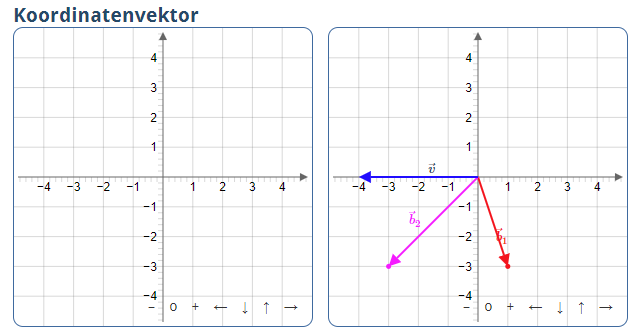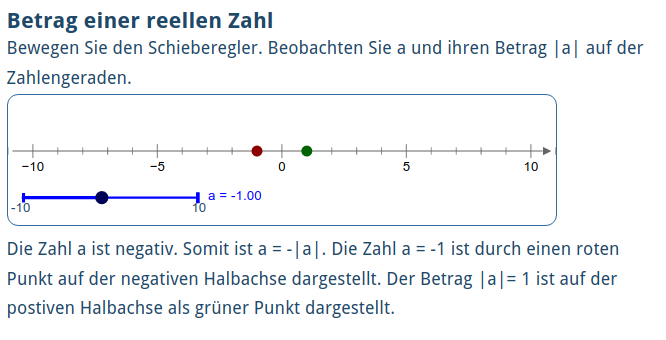Index

Working with MUMIE as author

• Initial steps:
• Articles:
• Problems:
• Programming with Python
• New Visualizations with JSXGraph
• Old Visualizations:
• Media Documents:

Working with MUMIE as teacher

Using MUMIE via plugin in local LMS

FAQ for examination lecturers

Overview

Go back to the old wiki

We revise and update this wiki. We apologize for the inconvenience this may cause you.

# JSX Visualizations

Caution: Generic JSX-Visualizations only work on servers supporting the new layout. So they currently are not supported in Mumie courses.
JSX-Visualizations using jsxgraph code can also be used in Mumie courses.

For Visualizations there are two new environments:
\begin{genericJSXVisualization}... \end{genericJSXVisualization} as well as
\begin{jsxgraph}... \end{jsxgraph}.
Both are using JSXGraph developped at the University of Bayreuth.
The former environment is more comfortable to the user as the code is written in LaTeX-style. In the latter
you can use actual javascript-code to create custom visualizations with the jsxgraph package.

Generic JSX-Visualizations can also be used in combination with problems. This means,
they can fetch values of number and function variables from the problem, and pass values of number and function variables to the problem as solutions to answers of type graphics.number and graphics.function.

# Generic JSX-Visualizations in articles

If you are familiar with the generic GWT-Visualizations (used in articles before), you can read the
explanation on changes with respect to genericGWTVisualization.

Here, we present a full explanation of how to create JSX-visualizations.

## General structure

Example:

12345678910111213141516171819202122232425262728293031\begin{visualizationwrapper}......\begin{genericJSXVisualization}{jsxviz}    \title{Coordinates of a point}  \begin{variables}     \point[editable]{p1}{real}{1,0}    % the point can be dragged as it is set to editable.    \number{px}{real}{p1[x]}    \number{py}{real}{p1[y]}  \end{variables}   \label{p1}{P}      \color{p1}{DARKRED}   \text{In der Grafik sehen sie den Punkt $P=\var{p1}$.}   \begin{canvas}    \plotSize{200,200}    \plotLeft{-10}    \plotRight{10}    \plot[coordinateSystem,showPointCoords]{p1}  \end{canvas}   \text{Die $x$-Koordinaten des Punktes ist $\var{px}$,\\ die $y$-Koordinate ist $\var{py}$.}    \end{genericJSXVisualization} ......\end{visualizationwrapper} 

The visualization environment starts with \begin{genericJSXVisualization}{_id_} and
ends with \end{genericJSXVisualization} where you can choose any string as _id_.
For enabling visualizations, they must lie in an environment \begin{visualizationwrapper},\end{visualizationwrapper}.
However, there is only one wrapper for all visualizations of an article, and arbitrary text components
of articles can also be inside this environment.

In contrast to text components of articles, a problem environment must not be inside the visualizationwrapper.

The components of the visualization are:

• a title given by \title{...},
• the definition of objects/variables between \begin{variables} and \end{variables},
• possible definitions of labels, colors and transparencies for the objects,
• arbitrary number of text-blocks (maybe none) given by \text{...},
• arbitrary number of canvases (at least one!) whose properties are given between \begin{canvas} and \end{canvas}.

The order in which the text-blocks and canvases appear in the code determines the order in which
they are shown later.

Two or more canvases can be displayed next to each other by using the canvasRow environment.

1234567891011121314151617181920\begin{genericJSXVisualization}{viz}    ...    \begin{canvasRow}           \begin{canvas}            \plotSize{300,300}            \plotLeft{-5}            \plotRight{5}            \plot[coordinateSystem]{vbvec}          \end{canvas}           \begin{canvas}            \plotSize{300,300}            \plotLeft{-5}            \plotRight{5}            \plot[coordinateSystem]{b1vec,b2vec,b1,b2,vvec,l1b1vec,l2b2vec}            \snapToGrid{0.1,0.1}        \end{canvas}    \end{canvasRow}    ... \end{genericJSXVisualization}In addition, there are environments tabs and incremental available.

The following example illustrates how it works. More details are given later.

1234567891011121314151617181920212223242526272829303132333435\begin{genericJSXVisualization}{viz}  \title{Betrag einer reellen Zahl}    \begin{variables}      \slider{s}{real}{-1}{-10}{10}      % slider with name s, initial value -1 and range [-10,10]     \number{a}{rational}{s}            % a rational number with name a and same value as s.     \number{b}{rational}{|a|}          % a rational number with name b and value equal to |a|.      \point{p1}{real}{a,0}              % non-editable point p1 on the x-axis with x-coordinate equal to a                         \point{p2}{real}{b,0}              % non-editable point p1 on the x-axis with x-coordinate equal to b \end{variables}  \label{s}{a}    \color{p1}{DARKRED} \color{p2}{DARKGREEN}  \text{Bewegen Sie den Schieberegler. Beobachten Sie $a$         und ihren Betrag  $|a|$ auf der Zahlengeraden.}  \begin{canvas}     \plotSize{500,120}     \plotLeft{-11}     \plotRight{11}     \plotBottom{-3}     \plot[numberLine, noToolbar]{p1,p2,s}      \end{canvas}  \text{ \IFELSE{s >= 0}{           Die Zahl $a$ is positiv oder Null. Somit          ist $a=|a|$ und auf der Zahlengeraden durch einen grünen Punkt dargestellt.        }{          Die Zahl $a$ ist negativ. Somit ist  $a = -|a|$. Die Zahl $a = \var{a}$ ist durch einen roten Punkt           auf der negativen Halbachse dargestellt. Der Betrag $|a|=\var{b}$ ist auf der postiven Halbachse als grüner Punkt dargestellt.        } }    \end{genericJSXVisualization}In the variables environment, there are five variables. The numbers and the points can not be changed directly,
as the optional editable flag is not set. However, when the value of the slider is changed the values of the

The label-command \label{s}{a} causes that a=-1.00 is displayed next to the slider, and the color-commands
change the default colors of the points _p1_ and _p2_.

Tip: Let's say your label is a formula like x^2 and you would like that it is rendered as a math formula instead of plain text. You can achieve that with the following syntax: \label{s}{\$x^2\$}

About the commands in the canvas environment:

• \plotSize determines the maximum size of the canvas in pixel (it is shrinked if the screen is too small),
• \plotLeft and \plotRight determine the lower and upper bound of the x-axis,
• \plotBottom determines the lower bound of the y-axis (the upper bound is then computed to fit),
• \plot determines which variables are displayed in the canvas (here _p1_, _p2_ and _s_), as well as sets some
attributes. In this case, that a number line is shown and that the toolbar is hidden.

The final text part contains an if-clause which shows either the first part or the second part depending on whether
the value of the slider _s_ is greater or equal to zero or not.

## Create visualization content

Under the following links you get detailed information on how to create different parts of the visualization content.

# Using Visualizations with Problems

Generic JSX-Visualizations can be used with problems, too.
The syntax of the visualization is the same as when used in articles.
However, there are some additional commands so that the visualization can interact with the problem.

## Getting variables from the problem

In the visualization you can use the variable-types question and problem with syntax:
\question{questionnr}{varname}{field} and \problem{varname}{field}.
The visualization then fetches the value of the variable varname from the specified question or problem,
and you can use that variable as any other variable.

There are some restrictions/issues that you have to take into account:

• The value of the question variable or problem variable has to be a number or a function in at most one indeterminate. Other formats are not supported.
• The visualization treats that variable as a number or a function depending on whether the value contains an indeterminate, irrespective of the variable type of the variable in the problem.
• As for most other variables, you can set an optional editable-flag to make the variable editable in text,
\question[editable]{questionnr}{varname}{field}.

Example:

1234567891011121314151617181920212223242526272829303132333435363738394041424344454647484950515253545556\usepackage{mumie.genericproblem}\begin{visualizationwrapper}\begin{genericJSXVisualization}    \begin{variables}        \question{1}{f}{real}  % the variable f will be fetched from question 1    \end{variables}     \begin{canvas}        \plotSize{250,250}        \plotLeft{-4}        \plotRight{4}        \plot[coordinateSystem]{f}    \end{canvas} \end{genericJSXVisualization} \end{visualizationwrapper} \begin{problem}         \begin{variables}        \randint{c}{1}{3}  % integer in the range [-3,3]        \begin{switch}          \begin{case}{c=1}            \function{f}{2*x+1}          \end{case}          \begin{case}{c=2}            \function{f}{2*x^2+1}          \end{case}          \begin{default}            \function{f}{|2*x+1|}          \end{default}        \end{switch}    \end{variables}   \begin{question}   \text{To what kind of function does the displayed graph belong.}     \begin{answer}        \type{mc.unique}         \begin{choice}            \text{Linear function}            \solution{compute}            \checkCorrect{c=1}        \end{choice}        \begin{choice}            \text{Quadratic function}            \solution{compute}            \checkCorrect{c=2}        \end{choice}        \begin{choice}            \text{Neither nor.}            \solution{compute}            \checkCorrect{c=3}        \end{choice}     \end{answer}             \end{question}     \end{problem} 

## Passing values of variables to the problem as answers

For passing a variable having a number value (type number, slider, segment or polygon) or
a function value (type function) as answer to the problem, one uses the attribute \answer{varname}{questionnr,answernr} (see example below)
in the same way as one defines colors or labels for variables.

In the problem, the answer-type of the specific answer has to be set to graphics.number or graphics.function.
The correction of answers of type graphics.number is done in the same way as for
input.number, and the correction of answers of type graphics.function is done in the same way as for input.function.
The only difference is that the student's solution is not given by input, but is passed to the problem from the visualization.

Example: (complete example at https://miau.mumie.net/web-miau/editor/content%2Fexamples%2FgraphicsNumber%2Fprb_exampleGraphicsNumber.src.tex )

1234567891011121314151617181920212223242526272829303132333435363738394041424344454647484950515253\usepackage{mumie.genericproblem}\begin{visualizationWrapper}\begin{genericJSXVisualization}    \begin{variables}        \question{1}{f}{real}  % the variable f will be fetched from question 1        \randint{a}{-3}{0}        \randint{b}{-2}{0}        \point[editable]{s}{real}{a,b}        \number{sx}{real}{s[x]}   % the x-coordinate of point s        \number{sy}{real}{s[y]}   % the y-coordinate of point s    \end{variables}\color{s}{RED}\label{s}{S}\answer{sx}{1,1}  % sx is used as solution for question 1, answer 1\answer{sy}{1,2}  % sy is used as solution for question 1, answer 2     \begin{canvas}        \plotSize{540,350}        \plotLeft{-4}        \plotRight{4}        \plot[coordinateSystem]{f,s}    \end{canvas} \end{genericJSXVisualization} \end{visualizationwrapper} \begin{problem}        \begin{question}    \begin{variables}        \randrat{x0}{1}{6}{2}{2} % half-integer in the range [1/2, 6/2].        \randint{b}{-3}{3}  % integer in the range [-3,3]        \number{m}{1}        \function{f}{m*(x-x0)^2+b}    \end{variables}      \text{Drag the point $S$ to the angular point of the parabola shown above.}     \begin{answer}        \type{graphics.number}  % this is corrected like input.number, but the                                % student's answer is not given by input, but                                % is set by the visualization-command \answer{sx}{1,1}        \solution{x0}     \end{answer}               \begin{answer}        \type{graphics.number} % this is corrected like input.number, but the                               % student's answer is not given by input, but                               % is set by the visualization-command \answer{sy}{1,2}        \solution{b}     \end{answer}             \end{question}     \end{problem} 

# Visualizations using jsxgraph code

The javascript library JSXgraph can be used in Mumie. For instance, examples from https://jsxgraph.org/wiki/index.php/Category:Examples can be easily imported and used within Mumie articles or problems.

123456789\begin{jsxgraph}\jxgbox[400px][300px]{box1}\begin{code}  const board = JXG.JSXGraph.initBoard('box1', {boundingbox: [-5, 2, 5, -2]});  var p = board.create('point',[-3,1], {name:'P', size:3, face: 'o'});  var q = board.create('point',[1,-1], {name:'Q', size:3, face: '[]', fixed:true});  var l = board.create('line',[p,q], {name:'l'});\end{code}\end{jsxgraph} 
• The whole visualization has to be wrapped in the jsxgraph-environment \begin{jsxgraph} ... \end{jsxgraph}.
• \jxgbox creates a canvas in which the board can be drawn. Its parameters are
1. width of the canvas (optional, default is 500px)
2. height of the canvas (optional, default is 400px or same as width)
3. id of the jxgbox which is used for initialising the board in the 'JXG.JSXGraph.initBoard'-command.
• The whole javascript-code for the jsxgraph-visualization is written in the code-environment \begin{code} ... \end{code}.

## Using buttons

Buttons for events on the canvas can also be used.
The syntax is \button[id]{label}{action}.

• The optional parameter will be the id of the button.
• The first mandatory parameter is the label text of the button
• The second mandatory parameter describes the action when the button is clicked. It is given by the call of a function that has to be defined in the code-environment.

Example: (complete example at https://miau.mumie.net/web-miau/editor/content%2Fexamples%2Fvisualizations%2FusingJSXGraphCode%2Fart_buttons_2.src.tex )

123456789101112131415161718192021\begin{jsxgraph}\jxgbox[500px][300px]{box1}\button{Change Q}{changeQ(currDir)}\begin{code} var directions = ['oben', 'rechts', 'unten', 'links']; const board = JXG.JSXGraph.initBoard('box1', {boundingbox: [-5, 3, 5, -3]}); var p = board.create('point',[-3,1], {name:'P', size:3, face: 'o'}); var q = board.create('point',[1,-1], {name:'Q', size:3, face: '[]', fixed:true}); var l = board.create('line',[p,q], {name:'l'}); var currDir = 'oben'; function changeQ(direction) {  switch (direction) {   case 'oben': q.moveTo([q.X(),q.Y()+1]); currDir = 'rechts'; break;   case 'rechts': q.moveTo([q.X()+1,q.Y()]); currDir = 'unten'; break;   case 'unten': q.moveTo([q.X(),q.Y()-1]); currDir = 'links'; break;   case 'links': q.moveTo([q.X()-1,q.Y()]); currDir = 'oben'; break;   default: console.log('Unidentified direction');  } }\end{code}\end{jsxgraph}